4.7 Insertion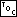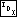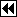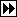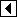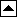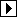Inserting new nodes into a binary search tree is easy. To start out, we work the same way as in a search, traversing the tree from the top down, as if we were searching for the item that we're inserting. If we find one, the item is already in the tree, and we need not insert it again. But if the new item is not in the tree, eventually we “fall off” the bottom of the tree. At this point we graft the new item as a child of the node that we last examined.

An example is in order. Consider this binary search tree:

Suppose that we wish to insert a new item, 7, into the tree. 7 is greater than 5, so examine 5's right child, 8. 7 is less than 8, so examine 8's left child, 6. 7 is greater than 6, but 6 has no right child. So, make 7 the right child of 6: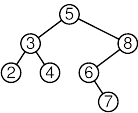We cast this in a form compatible with the abstract description as follows:

```32. <BST item insertion function 32> =
void **bst_probe (struct bst_table *tree, void *item) {
struct bst_node *p, *q; /* Current node in search and its parent. */
int dir;                /* Side of q on which p is located. */
struct bst_node *n;     /* Newly inserted node. */

assert (tree != NULL && item != NULL);

for (q = NULL, p = tree->bst_root; p != NULL; q = p, p = p->bst_link[dir])     {
int cmp = tree->bst_compare (item, p->bst_data, tree->bst_param);
if (cmp == 0)
return &p->bst_data;
dir = cmp > 0;
}

n = tree->bst_alloc->libavl_malloc (tree->bst_alloc, sizeof *p);
if (n == NULL)
return NULL;

tree->bst_count++;
n->bst_data = item;
if (q != NULL)
else     tree->bst_root = n;

return &n->bst_data;
}
```

This code is included in 29.

See also:  [Knuth 1998b], algorithm 6.2.2T; [Cormen 1990], section 13.3; [Bentley 2000], section 13.3; [Sedgewick 1998], program 12.7.

Exercises:

1. Explain the expression p = (struct bst_node *) &tree->bst_root. Suggest an alternative. [answer]

2. Rewrite bst_probe() to use only a single local variable of type struct bst_node **. [answer]

3. Suppose we want to make a new copy of an existing binary search tree, preserving the original tree's shape, by inserting items into a new, currently empty tree. What constraints are there on the order of item insertion? [answer]

4. Write a function that calls a provided bst_item_func for each node in a provided BST in an order suitable for reproducing the original BST, as discussed in Exercise 3. [answer]